Functions and CALL Routines

# YIELDP Function

Returns the yield-to-maturity for a periodic cash flow stream, such as a bond.
 Category: Financial

## Syntax

 YIELDP(A,c,n,K,k0,p)

### Arguments

A

specifies the face value.

 Range: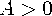c

specifies the nominal annual coupon rate, expressed as a fraction.

 Range: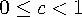n

specifies the number of coupons per year.

 Range: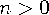and is an integer
K

specifies the number of remaining coupons from settlement date to maturity.

 Range: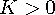and is an integer
k0

specifies the time from settlement date to the next coupon as a fraction of the annual basis.

 Range: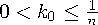p

specifies the price with accrued interest.

 Range: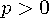The YIELDP function is based on the relationship
where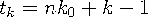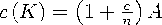The YIELDP function solves for y.

In the following example, the YIELDP function returns the yield-to-maturity of a bond that has a face value of 1000, an annual coupon rate of 0.01, 4 coupons per year, and 14 remaining coupons. The time from settlement date to next coupon date is 0.165, and the price with accrued interest is 800.

```data _null_;
y=yieldp(1000,.01,4,14,.165,800);
put y;
run;  ```

The value returned is 0.0775031248.Previous Page | Next Page | Top of Page